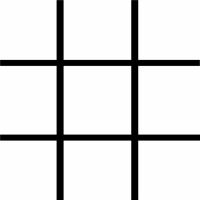# Tic-Tac-Toe Puzzle to place numbers

1,205.2K Views

You have to place all the digits from 1 to 9 without repeating any number in a tic tac toe board..
So, that the numbers should add up to 15 whether you add the numbers in each row, column or diagonally.i Have found out elements using C program. Ans is

4 | 9 | 2
———-
3 | 5 | 7
———-
8 | 1 | 6
Here is the code.

``````#include < stdio.h >
#include < conio.h >
int main() {
int size = 3;
int matrix; // = {{4,9,2},{3,5,7},{8,1,6}};
int row, column = 0;
int sum, sum1, sum2;
int flag = 0;
printf("nEnter matrix : ");
for (row = 0; row < size; row++) {
for (column = 0; column < size; column++)
scanf("%d", & matrix[row][column]);
}

printf("Entered matrix is : n");
for (row = 0; row < size; row++) {
printf("n");
for (column = 0; column < size; column++) {
printf("t%d", matrix[row][column]);
}
}

//For diagonal elements
sum = 0;
for (row = 0; row < size; row++) {
for (column = 0; column < size; column++) {
if (row == column)
sum = sum + matrix[row][column];
}
}

//For Rows
for (row = 0; row < size; row++) {
sum1 = 0;
for (column = 0; column < size; column++) {
sum1 = sum1 + matrix[row][column];
}
if (sum == sum1)
flag = 1;
else {
flag = 0;
break;
}
}

//For Columns
for (row = 0; row < size; row++) {
sum2 = 0;
for (column = 0; column < size; column++) {
sum2 = sum2 + matrix[column][row];
}
if (sum == sum2)
flag = 1;
else {
flag = 0;
break;
}
}

if (flag == 1)
printf("nMagic square");
else
printf("nNo Magic square");
return 0;
}
``````Superb (Y)

on 29th October 2015.code is not even compiling !

on 16th April 2016.